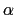Next: List of available colvar Up: Collective Variables Interface (Colvars) Previous: Performance a Colvars calculation   Contents   Index

# Collective variable components (basis functions)

Each colvar is defined by one or more components (typically only one). Each component consists of a keyword identifying a functional form, and a definition block following that keyword, specifying the atoms involved and any additional parameters (cutoffs, reference'' values, ...).

The types of the components used in a colvar determine the properties of that colvar, and which biasing or analysis methods can be applied. In most cases, the colvar returns a real number, which is computed by one or more instances of the following components:

• distance: distance between two groups;
• distanceZ: projection of a distance vector on an axis;
• distanceXY: projection of a distance vector on a plane;
• distanceInv: mean distance between two groups of atoms (e.g. NOE-based distance);
• angle: angle between three groups;
• dipoleAngle: angle between two groups and dipole of a third group;
• coordNum: coordination number between two groups;
• selfCoordNum: coordination number of atoms within a group;
• hBond: hydrogen bond between two atoms;
• rmsd: root mean square deviation (RMSD) from a set of reference coordinates;
• eigenvector: projection of the atomic coordinates on a vector;
• orientationAngle: angle of the best-fit rotation from a set of reference coordinates;
• orientationProj: cosine of orientationProj;
• spinAngle: projection orthogonal to an axis of the best-fit rotation from a set of reference coordinates;
• tilt: projection on an axis of the best-fit rotation from a set of reference coordinates;
• gyration: radius of gyration of a group of atoms;
• inertia: moment of inertia of a group of atoms;
• inertiaZ: moment of inertia of a group of atoms around a chosen axis;
• alpha:-helix content of a protein segment.
• dihedralPC: projection of protein backbone dihedrals onto a dihedral principal component.

Some components do not return scalar, but vector values. They can only be combined with vector values of the same type, except within a scripted collective variable.

• distanceVec: distance vector between two groups;
• distanceDir: unit vector parallel to distanceVec;
• cartesian: vector of atomic Cartesian coordinates;
• orientation: best-fit rotation, expressed as a unit quaternion.

In the following, all the available component types are listed, along with their physical units and the limiting values, if any. Such limiting values can be used to define lowerBoundary and upperBoundary in the parent colvar.

For each type of component, the available configurations keywords are listed: when two components share certain keywords, the second component simply references to the documentation of the first regarding that keyword. The keywords that are available for all types of components are listed at the end (see

SubsectionsNext: List of available colvar Up: Collective Variables Interface (Colvars) Previous: Performance a Colvars calculation   Contents   Index
vmd@ks.uiuc.edu